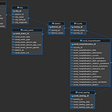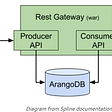# ClickHouse Window Functions — Current State of the Art

Window functions have long been a top feature request in ClickHouse. Thanks to excellent work by Alexander Kuzmenkov, ClickHouse now has experimental support, and users can begin to try them out. Naturally we did the same at Altinity. In fact, we tested the functionality extensively to find what currently works, what doesn’t, and what is not supported.

The result of this work was a set of tests that we have developed and added to ClickHouse repository. In addition to just writing tests, we have, as always, described the functionality that we test using requirements that help us understand and manage the process of testing each feature. In this article, we will take a comprehensive look at window functions, including an intro for new users, detailed syntax, and a summary of current limitations. For this article, we used the latest stable ClickHouse version 21.5.5.12.

Note: Our friends at Tinybird.co also blogged about window functions recently. You can expect more community articles and talks as this long-awaited feature becomes fully available in stable releases.

# Window What?

Window functions allow users to perform calculations on data rows based on some relation of these rows to the current row inside a so-called window. Therefore, with window functions you always have to keep the current row in mind. A simple example of using window functions is a calculation of a moving average. For example, we can easily calculate a moving average for the last four values in a series by using ROWS frame and applying the avg aggregate function over it.

`SELECT    number,    avg(number) OVER (ROWS BETWEEN 3 PRECEDING AND CURRENT ROW) AS mv4FROM values('number Int8', 1, 2, 3, 4, 5, 6, 7, 8)┌─number─┬─mv4─┐│      1 │   1 ││      2 │ 1.5 ││      3 │   2 ││      4 │ 2.5 ││      5 │ 3.5 ││      6 │ 4.5 ││      7 │ 5.5 ││      8 │ 6.5 │└────────┴─────┘`

Note that the output before number 4 is not valid as there is not enough data.

In addition the current row, there is also a concept of peers of the current row that applies to RANGE frames. Peers of the current row are the rows that fall into the same sort bucket if the ORDER BY clause is specified. We can observe this behavior in the following example.

`SELECT    number,    sum(number) OVER (ORDER BY number ASC RANGE BETWEEN CURRENT ROW AND CURRENT ROW)FROM numbers(1, 3)┌─number─┬─sum(number) OVER (ORDER BY number ASC RANGE BETWEEN CURRENT ROW AND CURRENT ROW)─┐│      1 │                                                                                1 ││      2 │                                                                                2 ││      3 │                                                                                3 │└────────┴──────────────────────────────────────────────────────────────────────────────────┘`

A detailed explanation might help. What we see above is that a window function is evaluated for each result row. Because we don’t have any PARTITION BY clause it means we only have one window. Now, because we have a RANGE frame clause it means for each row the window function evaluates a corresponding frame for that row. The frame is always sliding with the current row inside a window. The frame is specified as BETWEEN CURRENT ROW AND CURRENT ROW which means each frame only includes current row peers. Because we have an ORDER BY clause that assigns each value into its own sort bucket, then we have the case where current row is the same as current row peers. Therefore, each frame only includes the value of the current row which is passed to the sum function that in turn returns the value of the current row for each frame.

If no ORDER BY clause is specified, then all rows in the window partition are considered to be the peers of the current row as the query below illustrates this.

`SELECT    number,    sum(number) OVER (RANGE BETWEEN CURRENT ROW AND CURRENT ROW)FROM numbers(1, 3)┌─number─┬─sum(number) OVER (RANGE BETWEEN CURRENT ROW AND CURRENT ROW)─┐│      1 │                                                            6 ││      2 │                                                            6 ││      3 │                                                            6 │└────────┴──────────────────────────────────────────────────────────────┘`

What we can see is that now the window function returned the same result for each row. Why? Simple: we don’t have any ORDER BY clause and all rows in the result are considered to be the peers of the current row. While the frame is still sliding inside a window with each current row, the frame for each row always contains all the rows.

In general, a window can either include all rows in the result set or result set can be partitioned into separate windows using the PARTITION BY clause. So when no PARTITION BY clause is specified then all the rows fall into one big partition, also known as a window. Rows in each window then can be sorted using the ORDER BY clause. The window analogy comes in handy when you try to understand this functionality. Just create a mental image of a window that allows you to see either all the rows in the result set or just some chunk of them as the concept diagram below shows.

Now within a window you can also define a frame using a frame clause. The frame clause can either be defined in terms of the ROWS or the RANGE relationship to the current row. Nifty!

Of course, those who have already worked with window functions in either MySQL or PostgreSQL will find the syntax and functionality to be familiar and the basic explanation above unnecessary. However, if you are not familiar with window functions, then they can initially be hard to understand. Thus we find our first limitation of window functions in ClickHouse; the current ClickHouse documentation does not go into many details and so if you need to get familiar with window functions you have to use either documentation from PostgreSQL or MySQL. Some examples and explanations of the expected behavior can also be found in our requirements.

# The Mini Ninja-course For Defining Windows

Windows can either be named or inline defined using the OVER clause. Named windows are defined using WINDOW clause which is defined as

`WINDOW window_name AS (window_spec)    [, window_name AS (window_spec)] ..`

where window_name is a unique name for the specific window and window_spec is defined as

`window_spec:  	[partition_clause] [order_clause] [frame_clause]`

where the partition_clause is

`partition_clause:  	PARTITION BY expr [, expr] ...`

and the order_clause is

`order_clause:    ORDER BY expr [ASC|DESC] [, expr [ASC|DESC]] ...`

and the frame_clause is

`frame_clause:    {ROWS | RANGE } frame_extent`

and the frame_extent is

`frame_extent:    {frame_start | frame_between}`

and frame_start is

`frame_start: {    CURRENT ROW  | UNBOUNDED PRECEDING  | UNBOUNDED FOLLOWING  | expr PRECEDING  | expr FOLLOWING}`

and frame_between is

`frame_between:    BETWEEN frame_start AND frame_end`

and frame_end is

`frame_end: {    CURRENT ROW  | UNBOUNDED PRECEDING  | UNBOUNDED FOLLOWING  | expr PRECEDING  | expr FOLLOWING}`

oh, and again it is important to keep in mind that CURRENT ROW in the frame_start or frame_end for RANGE frames actually means peers of the current row. Again, the peers of the current row are any rows that fall into the same sort bucket defined by the ORDER BY clause, if any.

Let’s also not forget the OVER clause that is defined as

`OVER ()|(window_spec)|named_window`

where the special case of the OVER () is used to define a window that contains all the rows.

Don’t you love it? We do, but if you are not familiar with window functions then please resist the urge to hit something with a window or a window with a frame before digesting the summary above. It all makes sense once you start using the window functions! Also, don’t forget that some frame_between clauses might not be valid as you can define something that has a start before the end. Don’t do that unless you want to receive an error as we will see in the examples below.

# What About The Function Part?

After one or more windows are defined a function can be applied over them. There are two types of functions that we can apply to windows. First type are the so-called window native functions such as:

• row_number
• first_value
• last_value
• rank
• dense_rank
• lead (not currently supported but there is a workaround)
• lag (not currently supported but there is a workaround)

These functions can only be used over the windows. The second type are regular aggregate functions and ClickHouse supports applying over the windows all the standard aggregate functions such as min, max, avg etc. as well as ClickHouse specific aggreate functions such as argMin, argMax, avgWeighted etc.

Note that there are special aggregate functions such as initializeAggregation, aggregate function combinators, and parametric aggregate functions that we haven’t tested using over windows.

There are also some aggregate functions that were not easy to set up for testing as they required arrays so they were skipped. If you are interested, you can find the full list of aggregate functions tested with windows in tests/testflows/window_functions/tests/aggregate_funcs.py.

# Test Coverage

In addition to stateless window function tests, we at Altinity have also developed an additional test suite using our QA process. Given that window functions have a reference implementation, as a base, in our testing we have compared ClickHouse window functions’ behavior against PostgreSQL regression tests. We could not use these tests as-is, as there are enough differences between ClickHouse and PostgreSQL that require manual changes to test queries. Nonetheless, we have ported as many queries as we could to make sure ClickHouse’s window functions implementation returns the same results as in PostgreSQL.

We have also added additional test cases to provide better coverage for the ROWS and RANGE frame clauses including error corner cases. In addition, we have modified some tests to work when reference tables are sharded between a three-node cluster accessed using a Distributed table. This was needed because when the table is sharded the order of rows changes and to make test work with both distributed and non-distributed reference tables correct ORDER BY clauses had to be added.

Overall, with the requirements that we have defined, ClickHouse satisfies 93% of them. Here is a summary of the coverage report.

`SRS019 ClickHouse Window Functions  130 requirements (121 satisfied 93.1%, 7 unsatisfied 5.4%, 2 untested 1.5%)`

If you are interested, you can find our tests and requirements in the tests/testflows/window_functions folder inside ClickHouse’s GitHub repository.

# Potential Problems With Distributed Tables

For distributed tables, the current implementation forces window function calculation to be performed on the initiator node. This means that window function calculation is not spread out between shards but instead each shard has to send its data to the initiator to perform calculations. If the amount of data is large, then the initiator node can be left receiving and processing a lot of data with the potential of running out of memory.

Additionally, there are still bugs that need to be ironed out for distributed tables, for example issue 23902. This issue seems to be a regression from 21.5.5.12 in the latest master and hopefully can be resolved before the next official 21.6 release.

# The EXCLUDE Clause

The EXCLUDE clause is not supported and is not recognized by the parser. For example, the following query will produce an error.

`SELECT     sum(unique1) OVER (ROWS BETWEEN 2 PRECEDING AND 2 FOLLOWING EXCLUDE NO OTHERS) AS sum,    unique1,     four FROM tenk1 WHERE unique1 < 10Syntax error: failed at position 68 ('exclude')`

# The GROUPS Frame

The GROUPS frame is also not supported right now. The parser does recognize it and a not implemented error is returned. For example,

`SELECT    sum(unique1) OVER (ORDER BY four ASC GROUPS BETWEEN UNBOUNDED PRECEDING AND CURRENT ROW),    unique1,    fourFROM tenk1WHERE unique1 < 10Code: 48. DB::Exception: Received from localhost:9000. DB::Exception: Window frame 'GROUPS' is not implemented (while processing 'ORDER BY four ASC GROUPS BETWEEN UNBOUNDED PRECEDING AND CURRENT ROW').`

# No INTERVAL Support For Date And DateTime In RANGE Frames

The INTERVAL syntax is not supported for Date and DateTime types in the RANGE frame offsets. The workaround is just to specify the offset as a positive integer. For example, if you have a Date type column and you need a range frame being 1 year preceding and 1 year following the date of the current row then you can try to do the following.

`SELECT    sum(salary) OVER (ORDER BY enroll_date ASC RANGE BETWEEN 365 PRECEDING AND 365 FOLLOWING) AS sum,    salary,    enroll_dateFROM empsalaryORDER BY empno ASC`

The gotcha is that for Date type the integer value specifies the number of days and for DateTime type the value specifies the number of seconds. So, if you have DateTime column and you need 1 year interval you can try to do the following.

`SELECT    id,    f_timestamp,    first_value(id) OVER w AS first_value,    last_value(id) OVER w AS last_valueFROM datetimesWINDOW w AS (ORDER BY f_timestamp ASC RANGE BETWEEN 31536000 PRECEDING AND 31536000 FOLLOWING)ORDER BY id ASC`

The problem with the workarounds above is that it really does not work well in practice as calculation of date time intervals is much more complex. A simple example below shows what happens when we try to add one year to 2020–02–28 using fixed number of days.

`SELECT toDate('2020-02-28') + 365┌─plus(toDate('2020-02-28'), 365)─┐│                      2021-02-27 │└─────────────────────────────────┘SELECT toDate('2020-02-28') + toIntervalYear(1)┌─plus(toDate('2020-02-28'), toIntervalYear(1))─┐│                                    2021-02-28 │└───────────────────────────────────────────────┘`

The obvious problem above is that the number of days in each year is not constant.

# Lack of lag(value, offset) And lag(value, offset) Functions

The current lack of proper support for lead(value, offset) and lag(value, offset) functions is also unfortunate. The workaround for lag(value, offset) is to use

`any(value) over (.... rows between <offset> preceding and <offset> preceding)`

`any(value) over (.... rows between <offset> following and <offset> following)`

Therefore, where instead of just using lead(ten), with the default value of offset being 1, you have to write the following:

`SELECT    any(ten) OVER (PARTITION BY four ORDER BY ten ASC ROWS BETWEEN 1 FOLLOWING AND 1 FOLLOWING) AS lead,    ten,    fourFROM tenk1WHERE unique2 < 10`

Oh, and don’t forget that if you have NULLs and you don’t want to get the default value instead of a proper NULL value you have to use anyOrNull function as follows:

`SELECT    anyOrNull(ten) OVER (PARTITION BY four ORDER BY ten ASC ROWS BETWEEN 1 FOLLOWING AND 1 FOLLOWING) AS lead,    ten,    fourFROM tenk1WHERE unique2 < 10`

# Expressions Involving Window Functions

Expressions using window functions is also currently not supported. To illustrate, you can have a query as follows.

`SELECT count(*) OVER (PARTITION BY four ORDER BY ten ASC) + sum(hundred) OVER (PARTITION BY four ORDER BY ten ASC) AS cntsumFROM tenk1WHERE unique2 < 10`

If you do try it, you will get a very long error message.

`Code: 47. DB::Exception: Received from localhost:9000. DB::Exception: Unknown identifier: count() OVER (PARTITION BY four ORDER BY ten ASC); there are columns: less(unique2, 10), 10, 10, unique2, four, ten, hundred: While processing count() OVER (PARTITION BY four ORDER BY ten ASC) + sum(hundred) OVER (PARTITION BY four ORDER BY ten ASC) AS cntsum`

# RANGE Frame With Named Window

The RANGE frame with named window can’t be used. For instance, if you define a named window and you try to use it with RANGE frame it will not work.

`SELECT     * FROM (    SELECT         sum(unique1) OVER (w range BETWEEN CURRENT ROW AND UNBOUNDED FOLLOWING) AS sum,        unique1,         four     FROM tenk1     WHERE unique1 < 10     WINDOW w AS (order by four))ORDER BY unique1`

You instead get a lengthy syntax error.

`Code: 62. DB::Exception: Syntax error: failed at position 42 ('w'): w range between current row and unbounded following) AS sum,unique1, four FROM tenk1 WHERE unique1 < 10 WINDOW w AS (order by four)) ORDER BY unique1 FORMAT Tab. Expected one of: GROUPS, ClosingRoundBracket, ORDER BY, PARTITION BY, ROWS, RANGE, token`

# RANGE Frame Data Types And Offsets

The RANGE frame offsets also work only for floats and integer data types. Nullable or other data types are not supported. So, if you try using a Decimal column in the RANGE frame as below

`SELECT    id,    f_numeric,    first_value(id) OVER w AS first_value,    last_value(id) OVER w AS last_valueFROM numericsWINDOW w AS (ORDER BY f_numeric ASC RANGE BETWEEN 1 PRECEDING AND 1 FOLLOWING)`

you will be reminded that Decimal type is not supported with RANGE frame.

`DB::Exception: The RANGE OFFSET frame for 'DB::ColumnDecimal<DB::Decimal<long> >' ORDER BY column is not implemented`

Also, RANGE frame is not supported for Nullable columns. The example query below

`SELECT    number,    sum(number) OVER (ORDER BY number ASC RANGE BETWEEN CURRENT ROW AND 1 FOLLOWING) AS sumFROM values('number Nullable(Int8)', 1, 1, 2, 3, NULL)`

will return the following error.

`DB::Exception: The RANGE OFFSET frame for 'DB::ColumnNullable' ORDER BY column is not implemented`

Additionally, you can’t use a sub-query for offsets. If you have a query like

`SELECT     sum(unique1) OVER (ORDER BY unique1 ROWS (SELECT unique1 FROM tenk1 ORDER BY unique1 LIMIT 1) + 1 PRECEDING) AS sum,    unique1 FROM tenk1 WHERE unique1 < 10`

you will also get a syntax error.

`DB::Exception: Syntax error: failed at position 49 ('('): (SELECT unique1 FROM tenk1 ORDER BY unique1 LIMIT 1) + 1 PRECEDING) AS sum, unique1 FROM tenk1 WHERE unique1 < 10`

Similarly, column values can’t be used in range offsets. Thus, the following query will not work.

`SELECT     any(ten) OVER (PARTITION BY four ORDER BY ten ROWS BETWEEN four PRECEDING AND four PRECEDING) AS lag,    ten,    fourFROM tenk1 WHERE unique2 < 10`

Instead, you will again get a syntax error.

`DB::Exception: Syntax error: failed at position 67 ('four'): four PRECEDING AND four PRECEDING) AS lag , ten, four FROM tenk1 WHERE unique2 < 10`

# RANGE Frame Offsets Must Be Positive

Don’t forget that negative offsets can’t be used. Therefore, if you try to execute a query that has a negative offset as below

`SELECT    number,    sum(number) OVER (ORDER BY number ASC RANGE BETWEEN CURRENT ROW AND -1 FOLLOWING) AS sumFROM values('number Float64', 1, 1, 2)`

you will get the following error.

`DB::Exception: Window frame start offset must be nonnegative, -1 given`

# ORDER BY Clause Required For RANGE Frame

When you specify a RANGE frame the ORDER BY clause is required. In comparison, for the ROWS frame it is not. For example, if you use RANGE frame without ORDER BY clause like so

`SELECT    number,    sum(number) OVER (RANGE BETWEEN 1 FOLLOWING AND 2 FOLLOWING) AS sumFROM values('number Float64', 1, 1, 2)`

you will get an error.

`DB::Exception: The RANGE OFFSET window frame requires exactly one ORDER BY column, 0 given`

Note that the ORDER BY clause can use only one column. If we modify the query above and try to specify more than one column then you will receive an error.

`SELECT    number,    sum(number) OVER (ORDER BY number ASC, number ASC RANGE BETWEEN 1 FOLLOWING AND 2 FOLLOWING) AS sumFROM values('number Float64', 1, 1, 2)DB::Exception: The RANGE OFFSET window frame requires exactly one ORDER BY column, 2 given`

# Frame Range Must Be Valid

Not all frame ranges that can be defined are valid. Any frame that has start offset which is before the end offset is not valid. For example, this query

`SELECT    number,    sum(number) OVER (ORDER BY number ASC RANGE BETWEEN 2 FOLLOWING AND 1 FOLLOWING) AS sumFROM values('number Float64', 1, 1, 2)`

will return an error.

`DB::Exception: Received from localhost:9000. DB::Exception: Frame start offset 2 FOLLOWING does not precede the frame end offset 1 FOLLOWING`

# RANGE Frame Corner Case

There is a corner case for RANGE frame that will not work as expected. For example,

`SELECT    number,    sum(number) OVER (ORDER BY number ASC RANGE BETWEEN 0 FOLLOWING AND CURRENT ROW) AS sumFROM values('number Int8', 1, 1, 2, 3)`

seems like a valid query with a valid range where one would expect that the 0 FOLLOWING start of the frame would be treated as the CURRENT ROW. That is not the case, and instead you will get the following error.

`DB::Exception: Window frame 'RANGE BETWEEN 0 FOLLOWING AND CURRENT ROW' is invalid`

To fix this just use CURRENT ROW as the start frame instead. The fun part is that if you say 0 PRECEDING for the start frame it will work and the result will be the same as if you specified RANGE BETWEEN CURRENT ROW AND CURRENT ROW.

`SELECT    number,    sum(number) OVER (ORDER BY number ASC RANGE BETWEEN 0 PRECEDING AND CURRENT ROW) AS sumFROM values('number Int8', 1, 1, 2, 3)┌─number─┬─sum─┐│      1 │   2 ││      1 │   2 ││      2 │   2 ││      3 │   3 │└────────┴─────┘`

# Conclusion

In this article, we have provided a summary of the syntax to define SQL windows and frames in ClickHouse. Along with the syntax we have given numerous examples of queries. We have highlighted the testing that we at Altinity have performed to ensure that window functions behave correctly. We also looked at unsupported features and the corresponding workarounds as well as other small things that you might run into when using window functions.

Overall, windows functions, while still in experimental stage, are maturing very fast. We invite you to use this exciting new feature in your work with ClickHouse and report any issues that you find on GitHub.

Originally published on the Altinity blog on May 28, 2021.

--

--

--

## More from Altinity

The go-to resource to optimize ClickHouse performance, covering best practices, tips, tutorials from ClickHouse experts, community members, developers, data engineers, and more.

## Helium Mapper & Cargo MicroPython LoRaWAN Node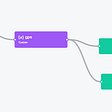## How to rule the chaos with Apache Flink?## How to hide Password / API Key in Python Script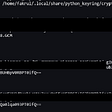## Hard vs Soft delete user data — forget-me or forget-me-not???## I Love My Watson Chatbot — How Do I Make Changes To It?## Practical Hints on Choosing Between FAT and NTFS## Best IDE to use for a Java Dev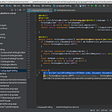## AltinityDB

Altinity is the leading enterprise provider for ClickHouse — a fast open-source column-store analytic database. Now on Cloud: altinity.com/cloud-database

## More from Medium